Read a noise covariance from a FIF file.

Parameters:
fnamepath-like

The path-like of file containing the covariance matrix. It should end with `-cov.fif` or `-cov.fif.gz`.

verbose

Control verbosity of the logging output. If `None`, use the default verbosity level. See the logging documentation and `mne.verbose()` for details. Should only be passed as a keyword argument.

Returns:
cov`Covariance`

The noise covariance matrix.

## Examples using `mne.read_cov`#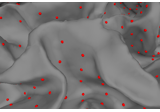The role of dipole orientations in distributed source localization

The role of dipole orientations in distributed source localization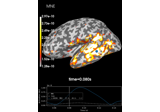Computing various MNE solutions

Computing various MNE solutions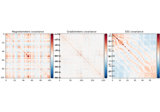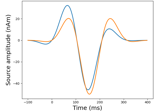Generate simulated evoked data

Generate simulated evoked data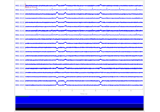Simulate raw data using subject anatomy

Simulate raw data using subject anatomy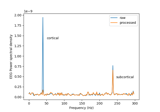Cortical Signal Suppression (CSS) for removal of cortical signals

Cortical Signal Suppression (CSS) for removal of cortical signals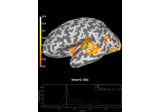Decoding source space data

Decoding source space data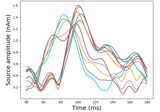Source localization with a custom inverse solver

Source localization with a custom inverse solver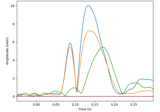Compute a sparse inverse solution using the Gamma-MAP empirical Bayesian method

Compute a sparse inverse solution using the Gamma-MAP empirical Bayesian method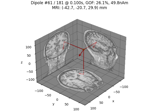Compute sparse inverse solution with mixed norm: MxNE and irMxNE

Compute sparse inverse solution with mixed norm: MxNE and irMxNE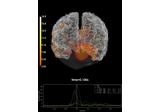Compute MNE inverse solution on evoked data with a mixed source space

Compute MNE inverse solution on evoked data with a mixed source space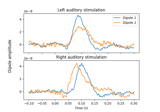Computing source timecourses with an XFit-like multi-dipole model

Computing source timecourses with an XFit-like multi-dipole model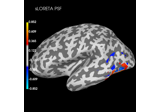Plot point-spread functions (PSFs) and cross-talk functions (CTFs)

Plot point-spread functions (PSFs) and cross-talk functions (CTFs)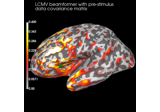Compute cross-talk functions for LCMV beamformers

Compute cross-talk functions for LCMV beamformers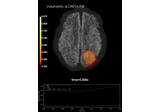Plot point-spread functions (PSFs) for a volume

Plot point-spread functions (PSFs) for a volume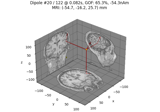Compute Rap-Music on evoked data

Compute Rap-Music on evoked data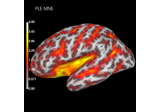Compute spatial resolution metrics in source space

Compute spatial resolution metrics in source space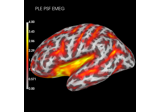Compute spatial resolution metrics to compare MEG with EEG+MEG

Compute spatial resolution metrics to compare MEG with EEG+MEG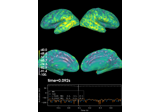Computing source space SNR

Computing source space SNR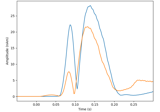Compute MxNE with time-frequency sparse prior

Compute MxNE with time-frequency sparse priorCompute Trap-Music on evoked data

Compute Trap-Music on evoked data